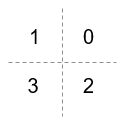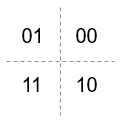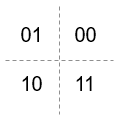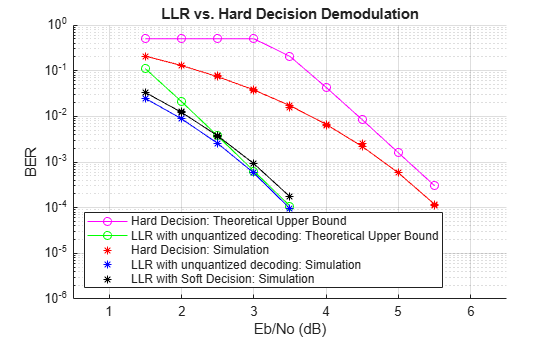# comm.QPSKDemodulator

Demodulate using QPSK method

## Description

The `comm.QPSKDemodulator` object demodulates a signal that was modulated using the quadrature phase shift keying (QPSK) method. The input is a baseband representation of the modulated signal.

To demodulate a signal that was modulated using the QPSK method:

1. Create the `comm.QPSKDemodulator` object and set its properties.

2. Call the object with arguments, as if it were a function.

## Creation

### Syntax

``qpskdemod = comm.QPSKDemodulator``
``qpskdemod = comm.QPSKDemodulator(Name=Value)``
``qpskdemod = comm.QPSKDemodulator(phase=Name,Value)``

### Description

``qpskdemod = comm.QPSKDemodulator` creates a System object™ to demodulate input QPSK signals.`

example

````qpskdemod = comm.QPSKDemodulator(Name=Value)` sets properties using one or more name-value arguments. For example, `DecisionMethod="Hard decision"` specifies demodulation using the hard-decision method.```

example

````qpskdemod = comm.QPSKDemodulator(phase=Name,Value)` sets the `PhaseOffset` property to `phase`, and optional name-value arguments. Specify `phase` in radians.```

## Properties

expand all

Unless otherwise indicated, properties are nontunable, which means you cannot change their values after calling the object. Objects lock when you call them, and the `release` function unlocks them.

If a property is tunable, you can change its value at any time.

Phase of the zeroth point in the constellation in radians, specified as a scalar.

Example: `PhaseOffset=0` aligns the QPSK signal constellation points on the axes {(1,0), (0,j), (-1,0), (0,-j)}.

Data Types: `double`

Option to output data as bits, specified as a logical `0` (`false`) or `1` (`true`).

• Set this property to `false` to output symbols as integer values in the range [`0`, `3`] with length equal to the input data vector length.

• Set this property to `true` to output a column vector of bit values with length equal to twice the input data vector length.

Data Types: `logical`

Symbol encoding mapping of constellation bits, specified as `'Gray'` or `'Binary'`.

SettingConstellation Mapping for IntegersConstellation Mapping for BitsComment

`Gray`Map symbols using Gray-coded ordering.

`Binary`Map symbols using natural binary-coded ordering. The signal constellation maps to the complex value ```ej(PhaseOffset + (2πm/4))```, where `m` is an integer in the range [0, 3].

Demodulation decision method, specified as `'Hard decision'`, `'Log-likelihood ratio'`, or ```'Approximate log-likelihood ratio'```. When you set the `BitOutput` property to `false`, the object always performs hard-decision demodulation.

#### Dependencies

To enable this property, set the `BitOutput` property to `true`.

Source of noise variance, specified as `'Property'` or `'Input port'`.

#### Dependencies

To enable this property, set the `BitOutput` property to `true` and the `DecisionMethod` property to `'Log-likelihood ratio'` or ```'Approximate log-likelihood ratio'```.

Noise variance, specified as a positive scalar.

Tunable: Yes

#### Tips

The exact LLR algorithm computes exponentials using finite precision arithmetic. For computations involving very large positive or negative magnitudes, the exact LLR algorithm yields:

• `Inf` or `-Inf` if the noise variance is a very large value

• `NaN` if the noise variance and signal power are both very small values

The approximate LLR algorithm does not compute exponentials. You can avoid `Inf`, `-Inf`, and `NaN` results by using the approximate LLR algorithm.

#### Dependencies

To enable this property, set the `BitOutput` property to `true`, the `DecisionMethod` property to `'Log-likelihood ratio'` or ```'Approximate log-likelihood ratio'```, and the `VarianceSource` property to `'Property'`.

Data Types: `double`

Data type of the output, specified as `'Full precision'`, `'Smallest unsigned integer'`, `'double'`, `'single'`, `'int8'`, `'uint8'`, `'int16'`, `'uint16'`, `'int32'`, or `'uint32'`,`'logical'`.

• When the input data type is single or double precision and you set the `BitOutput` property to `true`, the `DecisionMethod` property to `'Hard decision'`, and the `OutputDataType` property to `'Full precision'`, the output has the same data type as that of the input.

• When the input data is of a fixed-point type, the output data type behaves as if you had set the `OutputDataType` property to `'Smallest unsigned integer'`.

• When you set `BitOutput` to `true` and the `DecisionMethod` property to ```'Hard Decision'```, then `'logical'` data type is a valid option.

• When you set the `BitOutput` property to `true` and the `DecisionMethod` property to ```'Log-likelihood ratio'``` or `'Approximate log-likelihood ratio'`, the output data type is the same as that of the input and the input data type must be single or double precision.

#### Dependencies

To enable this property, set the `BitOutput` property to `false` or set the `BitOutput` property to `true` and the `DecisionMethod` property to `'Hard decision'`.

Fixed-Point Properties

Data type of the derotate factor, specified as ```'Same word length as input'``` or `'Custom'`. The object uses the derotate factor in the computations only when the input signal is a fixed-point type and the `PhaseOffset` property has a value that is not an even multiple of π/4.

#### Dependencies

To enable this property, set the `BitOutput` property to `false` or set the `BitOutput` property to `true` and the `DecisionMethod` property to `'Hard decision'`.

Fixed-point data type of the derotate factor, specified as an unscaled `numerictype` (Fixed-Point Designer) object with a `Signedness` of `Auto`.

#### Dependencies

To enable this property, set the `DerotateFactorDataType` property to `'Custom'`.

Data Types: `numerictype object`

## Usage

### Syntax

``y = qpskdemod(x)``
``y = qpskdemod(x,var)``

### Description

example

````y = qpskdemod(x)` applies QPSK demodulation to the input signal and returns the demodulated signal.```
````y = qpskdemod(x,var)` uses soft decision demodulation and noise variance `var`. This syntax applies when you set the `BitOutput` property to `true`, the `DecisionMethod` property to `'Approximate log-likelihood ratio'` or ```'Log-likelihood ratio'```, and the `VarianceSource` property to `'Input port'`.```

### Input Arguments

expand all

QPSK-modulated signal, specified as a scalar or column vector.

#### Dependencies

The object accepts inputs with a signed integer data type or signed fixed point (`sfi` (Fixed-Point Designer)) objects when you set the `BitOutput` property to `false` or you set the `DecisionMethod` property to `'Hard decision'` and the `BitOutput` property to `true`.

Data Types: `double` | `single` | `int` | `fi`

Noise variance, specified as a scalar.

#### Dependencies

To enable this argument, set the `VarianceSource` property to `'Input port'`, the `BitOutput` property to `true`, and the `DecisionMethod` property to `'Approximate log-likelihood ratio'` or ```'Log-likelihood ratio'```.

Data Types: `single` | `double`

### Output Arguments

expand all

Output signal, returned as a scalar or column vector. To specify whether the object outputs values as integers or bits, use the `BitOutput` property. To specify the output data type, use the `OutputDataType` property.

## Object Functions

To use an object function, specify the System object as the first input argument. For example, to release system resources of a System object named `obj`, use this syntax:

`release(obj)`

expand all

 `constellation` Calculate or plot ideal signal constellation
 `step` Run System object algorithm `release` Release resources and allow changes to System object property values and input characteristics `reset` Reset internal states of System object

## Examples

collapse all

Create a QPSK modulator.

`mod = comm.QPSKModulator;`

Determine the reference constellation points.

`refC = constellation(mod)`
```refC = 4×1 complex 0.7071 + 0.7071i -0.7071 + 0.7071i -0.7071 - 0.7071i 0.7071 - 0.7071i ```

Plot the constellation.

`constellation(mod)`Create a QPSK demodulator having phase offset set to `0`.

`demod = comm.QPSKDemodulator(0);`

Plot the reference constellation. The `constellation` method works for both modulator and demodulator objects.

`constellation(demod)`Create a QPSK modulator and demodulator pair that operate on bits.

```qpskModulator = comm.QPSKModulator('BitInput',true); qpskDemodulator = comm.QPSKDemodulator('BitOutput',true);```

Create an AWGN channel object and an error rate counter.

```channel = comm.AWGNChannel('EbNo',4,'BitsPerSymbol',2); errorRate = comm.ErrorRate;```

Generate random binary data and apply QPSK modulation.

```data = randi([0 1],1000,1); txSig = qpskModulator(data);```

Pass the signal through the AWGN channel and demodulate it.

```rxSig = channel(txSig); rxData = qpskDemodulator(rxSig);```

Calculate the error statistics. Display the BER.

```errorStats = errorRate(data,rxData); errorStats(1)```
```ans = 0.0100 ```

This example shows the BER performance improvement for QPSK modulation when using log-likelihood ratio (LLR) instead of hard-decision demodulation in a convolutionally coded communication link. With LLR demodulation, one can use the Viterbi decoder either in the unquantized decoding mode or the soft-decision decoding mode. Unquantized decoding, where the decoder inputs are real values, though better in terms of BER, is not practically viable. In the more practical soft-decision decoding, the demodulator output is quantized before being fed to the decoder. It is generally observed that this does not incur a significant cost in BER while significantly reducing the decoder complexity. We validate this experimentally through this example.

For a Simulink™ version of this example, see LLR vs. Hard Decision Demodulation in Simulink.

Initialization

Initialize simulation parameters.

```M = 4; % Modulation order k = log2(M); % Bits per symbol bitsPerIter = 1.2e4; % Number of bits to simulate EbNo = 3; % Information bit Eb/No in dB```

Initialize coding properties for a rate 1/2, constraint length 7 code.

```codeRate = 1/2; % Code rate of convolutional encoder constLen = 7; % Constraint length of encoder codeGenPoly = [171 133]; % Code generator polynomial of encoder tblen = 32; % Traceback depth of Viterbi decoder trellis = poly2trellis(constLen,codeGenPoly);```

Create a `comm.ConvolutionalEncoder` System object™ by using `trellis` as an input.

`enc = comm.ConvolutionalEncoder(trellis);`

Modulator and Channel

Create a `comm.QPSKModulator` and two `comm.QPSKDemodulator` System objects. Configure the first demodulator to output hard-decision bits. Configure the second to output LLR values.

```qpskMod = comm.QPSKModulator('BitInput',true); demodHard = comm.QPSKDemodulator('BitOutput',true,... 'DecisionMethod','Hard decision'); demodLLR = comm.QPSKDemodulator('BitOutput',true,... 'DecisionMethod','Log-likelihood ratio');```

The signal going into the AWGN channel is the modulated encoded signal. To achieve the required noise level, adjust the Eb/No for coded bits and multi-bit symbols. Calculate the $SNR$ value based on the ${E}_{b}/{N}_{o}$ value you want to simulate.

`SNR = convertSNR(EbNo,"ebno","BitsPerSymbol",log2(M),"CodingRate",codeRate);`

Viterbi Decoding

Create `comm.ViterbiDecoder` objects to act as the hard-decision, unquantized, and soft-decision decoders. For all three decoders, set the traceback depth to `tblen`.

```decHard = comm.ViterbiDecoder(trellis,'InputFormat','Hard', ... 'TracebackDepth',tblen); decUnquant = comm.ViterbiDecoder(trellis,'InputFormat','Unquantized', ... 'TracebackDepth',tblen); decSoft = comm.ViterbiDecoder(trellis,'InputFormat','Soft', ... 'SoftInputWordLength',3,'TracebackDepth',tblen);```

Calculating the Error Rate

Create `comm.ErrorRate` objects to compare the decoded bits to the original transmitted bits. The Viterbi decoder creates a delay in the decoded bit stream output equal to the traceback length. To account for this delay, set the `ReceiveDelay` property of the `comm.ErrorRate` objects to `tblen`.

```errHard = comm.ErrorRate('ReceiveDelay',tblen); errUnquant = comm.ErrorRate('ReceiveDelay',tblen); errSoft = comm.ErrorRate('ReceiveDelay',tblen);```

System Simulation

Generate `bitsPerIter` message bits. Then convolutionally encode and modulate the data.

```txData = randi([0 1],bitsPerIter,1); encData = enc(txData); modData = qpskMod(encData);```

Pass the modulated signal through an AWGN channel.

`[rxSig,noiseVariance] = awgn(modData,SNR);`

Before using a `comm.ViterbiDecoder` object in the soft-decision mode, the output of the demodulator needs to be quantized. This example uses a `comm.ViterbiDecoder` object with a `SoftInputWordLength` of 3. This value is a good compromise between short word lengths and a small BER penalty. Define partition points for 3-bit quantization.

```demodLLR.Variance = noiseVariance; partitionPoints = (-1.5:0.5:1.5)/noiseVariance;```

Demodulate the received signal and output hard-decision bits.

`hardData = demodHard(rxSig);`

Demodulate the received signal and output LLR values.

`LLRData = demodLLR(rxSig);`

Hard-decision decoding

Pass the demodulated data through the Viterbi decoder. Compute the error statistics.

```rxDataHard = decHard(hardData); berHard = errHard(txData,rxDataHard);```

Unquantized decoding

Pass the demodulated data through the Viterbi decoder. Compute the error statistics.

```rxDataUnquant = decUnquant(LLRData); berUnquant = errUnquant(txData,rxDataUnquant);```

Soft-decision decoding

Pass the demodulated data to the `quantiz` function. This data must be multiplied by `-1` before being passed to the quantizer, because, in soft-decision mode, the Viterbi decoder assumes that positive numbers correspond to 1s and negative numbers to 0s. Pass the quantizer output to the Viterbi decoder. Compute the error statistics.

```quantizedValue = quantiz(-LLRData,partitionPoints); rxDataSoft = decSoft(double(quantizedValue)); berSoft = errSoft(txData,rxDataSoft);```

Running Simulation Example

Simulate the previously described communications system over a range of Eb/No values by executing the simulation file `simLLRvsHD`. It plots BER results as they are generated. BER results for hard-decision demodulation and LLR demodulation with unquantized and soft-decision decoding are plotted in red, blue, and black, respectively. A comparison of simulation results with theoretical results is also shown. Observe that the BER is only slightly degraded by using soft-decision decoding instead of unquantized decoding. The gap between the BER curves for soft-decision decoding and the theoretical bound can be narrowed by increasing the number of quantizer levels.

This example may take some time to compute BER results. If you have the Parallel Computing Toolbox™ (PCT) installed, you can set `usePCT` to `true` to run the simulation in parallel. In this case, the file `LLRvsHDwithPCT` is run.

To obtain results over a larger range of Eb/No values, modify the appropriate supporting files. Note that you can obtain more statistically reliable results by collecting more errors.

```usePCT = false; if usePCT && license('checkout','Distrib_Computing_Toolbox') ... && ~isempty(ver('parallel')) LLRvsHDwithPCT(1.5:0.5:5.5,5); else simLLRvsHD(1.5:0.5:5.5,5); end```Appendix

The following functions are used in this example: